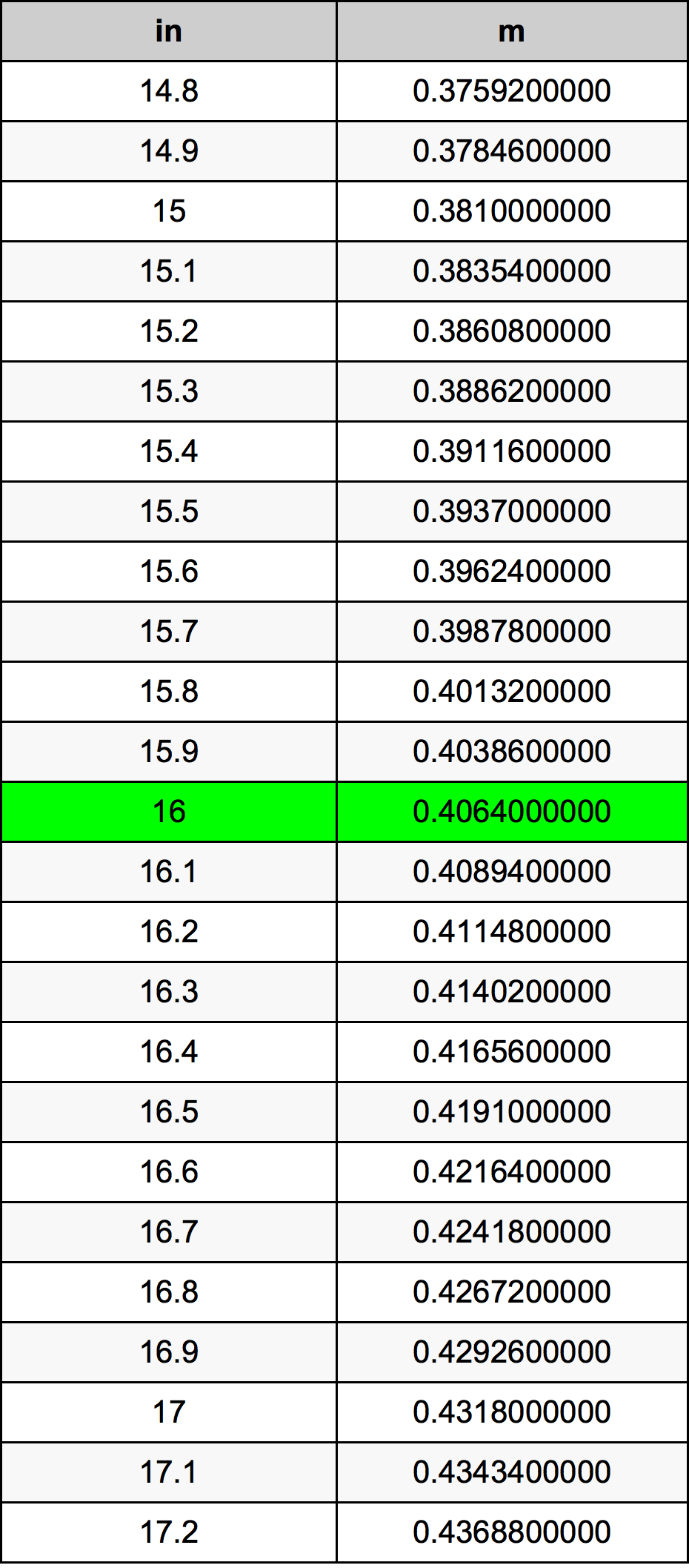Inches To Meters

# 16 in to m16 Inches to Meters

in
=
m

## How to convert 16 inches to meters?

 16 in * 0.0254 m = 0.4064 m 1 in
A common question is How many inch in 16 meter? And the answer is 629.921259843 in in 16 m. Likewise the question how many meter in 16 inch has the answer of 0.4064 m in 16 in.

## How much are 16 inches in meters?

16 inches equal 0.4064 meters (16in = 0.4064m). Converting 16 in to m is easy. Simply use our calculator above, or apply the formula to change the length 16 in to m.

## Convert 16 in to common lengths

UnitLengths
Nanometer406400000.0 nm
Micrometer406400.0 µm
Millimeter406.4 mm
Centimeter40.64 cm
Inch16.0 in
Foot1.3333333333 ft
Yard0.4444444444 yd
Meter0.4064 m
Kilometer0.0004064 km
Mile0.0002525253 mi
Nautical mile0.0002194384 nmi

## What is 16 inches in m?

To convert 16 in to m multiply the length in inches by 0.0254. The 16 in in m formula is [m] = 16 * 0.0254. Thus, for 16 inches in meter we get 0.4064 m.

## 16 Inch Conversion Table## Alternative spelling

16 in to Meter, 16 in in Meter, 16 Inches to m, 16 Inches in m, 16 in to m, 16 in in m, 16 Inches to Meter, 16 Inches in Meter, 16 Inches to Meters, 16 Inches in Meters, 16 in to Meters, 16 in in Meters, 16 Inch to m, 16 Inch in m